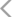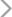﻿ 韩永辉_经典指标之倒锤线_砖家团_阿牛直播
• 综合
• 股票
• 板块
• 嘉宾
• 课程
• 基金
• 经理
• 说说
• 掌评
• 消息

经典指标之倒锤线

1、倒垂线主图：

M5:MA(C,5);

M10:MA(C,10);

M20:MA(C,20);

T:=BARSLAST(L>REF(L,1) AND REF(L,1)<REF(L,2));

SY:=H/MAX(C,O)*100;

XY:=MIN(C,O)/L*100;

BL:=SY/XY*100;

TJ:=BL>103.85 AND SY>105.05;

TJ1:=L>MAX(MAX(M5,M10),M20);

TJ2:=T=2 OR T=3;

XG:=TJ AND TJ1 AND TJ2;

DRAWICON(XG,L*0.95,1);

STICKLINE(XG,C,O,2,0),COLORYELLOW;

STICKLINE(XG,L,H,0,0),COLORYELLOW;

2、倒垂线副图：

M5:=MA(C,5);

M10:=MA(C,10);

M20:=MA(C,20);

T:=BARSLAST(L>REF(L,1) AND REF(L,1)<REF(L,2));

SY:=H/MAX(C,O)*100;

XY:=MIN(C,O)/L*100;

BL:=SY/XY*100;

TJ:=BL>103.85 AND SY>105.05;

TJ1:=L>MAX(MAX(M5,M10),M20);

TJ2:=T=2 OR T=3;

XG:=TJ AND TJ1 AND TJ2;

STICKLINE(XG,0,8,2,0),COLORYELLOW;

3、倒垂线选股：

M5:=MA(C,5);

M10:=MA(C,10);

M20:=MA(C,20);

T:=BARSLAST(L>REF(L,1) AND REF(L,1)<REF(L,2));

SY:=H/MAX(C,O)*100;

XY:=MIN(C,O)/L*100;

BL:=SY/XY*100;

TJ:=BL>103.85 AND SY>105.05;

TJ1:=L>MAX(MAX(M5,M10),M20);

TJ2:=T=2 OR T=3;

XG:TJ AND TJ1 AND TJ2;

﻿

• 点掌主题
• 点掌成长
• 点掌蓝筹

• 一周
• 一月
• 三月
• 六月
• 一年
• 创建以来
• 对标涨跌：

人气排行更多• 手机号码：

请输入手机号码，您的个人信息严格保密，请放心

• 图形验证码：

请输入右侧图形验证码

• 短信验证码：

请输入接收的短信验证码

获取短信验证码
X

X

（一）按照“后台实名、前台自愿”原则，对注册用户进行真实身份信息认证，不得向未认证真实身份信息的用户提供跟帖评论服务。

（二）建立健全用户信息保护制度，收集、使用用户个人信息应当遵循合法、正当、必要的原则，公开收集、使用规则，明示收集、使用信息的目的、方式和范围，并经被收集者同意。

（三）对新闻信息提供跟帖评论服务的，应当建立先审后发制度。

（四）提供“弹幕”方式跟帖评论服务的，应当在同一平台和页面同时提供与之对应的静态版信息内容。

（五）建立健全跟帖评论审核管理、实时巡查、应急处置等信息安全管理制度，及时发现和处置违法信息，并向有关主管部门报告。

（六）开发跟帖评论信息安全保护和管理技术，创新跟帖评论管理方式，研发使用反垃圾信息管理系统，提升垃圾信息处置能力；及时发现跟帖评论服务存在的安全缺陷、漏洞等风险，采取补救措施，并向有关主管部门报告。

（七）配备与服务规模相适应的审核编辑队伍，提高审核编辑人员专业素养。

（八）配合有关主管部门依法开展监督检查工作，提供必要的技术、资料和数据支持。• 10牛
• 30牛
• 50牛
• 其它